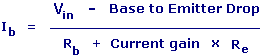BJT Transistor Bias Voltage Calculator Enter value and click on calculate. Result will be displayed.

 Enter your values: Base Bias Type: Series Resistor Voltage Divider VoltsRc = Collector Resistance Re = Emitter Resistance Vs = Supply Voltage Vc = Collector Voltage Ve = Emitter Voltage Vb = Base VoltageIc = Collector gain x Ib Ve = IC x Re Vb = Ve x Base to Emitter Drop Vc = Vs - IC x Rc If (Vin < Vb) then, Vc = Vs Ve = 0 Vb = Base to Emitter Drop Ic = 0 Ib = 0Rb = Base Resistance Vin = Input Voltage Rc = Collector Resistance Re = Emitter Resistance Vs = Supply Voltage Vc = Collector Voltage Ve = Emitter Voltage Vb = Base VoltageIc = Collector gain x Ib Ve = IC x Re Vb = Ve x Base to Emitter Drop Vc = Vs - IC x Rc If (Vc < Ve) then,Vc = Ve Vb = Ve + Base to Emitter DropBase Resistance (Rb): K Ohms Input Voltage (Vin): Volts Ra: K ohms Rb: K ohms Collector Resistance (Rc): K ohms Emitter Resistance (Re): K ohms Supply Voltage (Vs): Volts Current gain: Base to Emitter Drop: Volts Results: Collector Voltage (Vc): Volts Emitter Voltage (Ve): Volts Base Voltage (Vb): Volts Collector Voltage (Ic): mA Base Voltage (Ib): mA

 Web www.calculatoredge.com
 A Bipolar Junction Transistor (BJT) was invented in December 1947 at the Bell Telephone Laboratories by John Bardeen and Walter Brattain under the direction of William Shockley.

A Bipolar Junction Transistor is a solid-state device in which the current flow between two terminals (the collector and the emitter) is controlled by the amount of current that flows through a third terminal (the base).

A Bipolar Junction Transistor can be used for analog circuits, especially for very-high-frequency applications, such as radio-frequency circuits for wireless systems. Bipolar transistors can be combined with MOSFETs in an integrated circuit by using a BiCMOS process to create innovative circuits that take advantage of the best characteristics of both types of transistor.LINKSDISCLAIMERCONTACT US